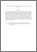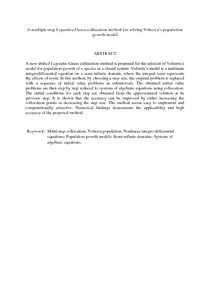# A multiple-step Legendre-Gauss collocation method for solving Volterra's population growth model

## Citation

Kajani, Majid Tavassoli and Maleki, Mohammad and Kilicman, Adem (2013) A multiple-step Legendre-Gauss collocation method for solving Volterra's population growth model. Mathematical Problems in Engineering, 2013. art. no. 783069. pp. 1-6. ISSN 1024-123X

## Abstract

A new shifted Legendre-Gauss collocation method is proposed for the solution of Volterra’s model for population growth of a species in a closed system. Volterra’s model is a nonlinear integrodifferential equation on a semi-infinite domain, where the integral term represents the effects of toxin. In this method, by choosing a step size, the original problem is replaced with a sequence of initial value problems in subintervals. The obtained initial value problems are then step by step reduced to systems of algebraic equations using collocation. The initial conditions for each step are obtained from the approximated solution at its previous step. It is shown that the accuracy can be improved by either increasing the collocation points or decreasing the step size. The method seems easy to implement and computationally attractive. Numerical findings demonstrate the applicability and high accuracy of the proposed method.Preview
PDF (Abstract)
A multiple.pdfView Item